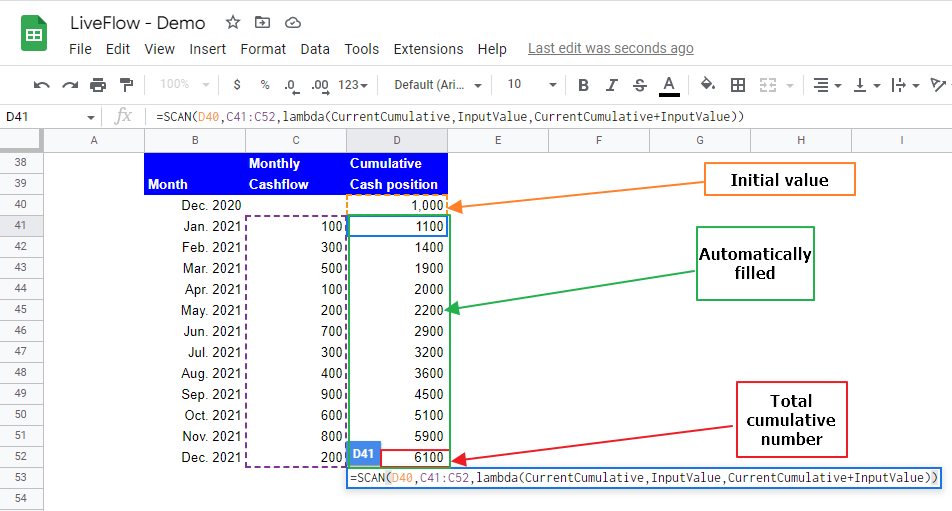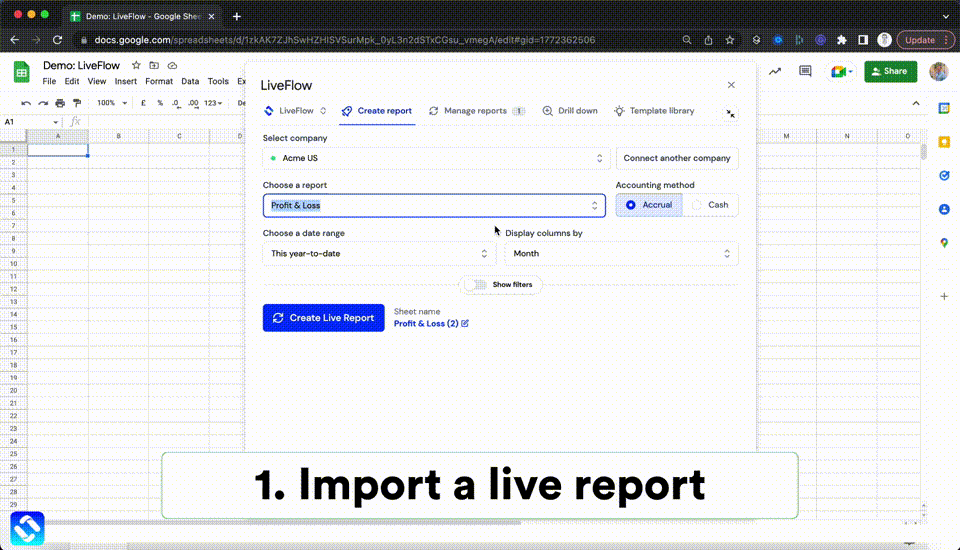# How to Use SCAN Function in Google Sheets

In this article, you will learn the SCAN formula and how it works in Google Sheets. This function is meant to be used with the LAMBDA function. The SCAN formula processes each value in a selected range or array as input values for the LAMBDA function in an accumulated way. The SCAN formula returns each application result (intermediate result) in cells, and you will see the total accumulated number in the last cell in the array. The output size is the same as the chosen area for the input values. If all assumptions are the same, the SCAN and REDUCE functions return the same total cumulative result. The difference is that the SCAN function shows all intermediate results leading to the final cumulative number, whereas the REDUCE function presents only the last cumulative number in a cell.

## How to insert the SCAN formula in Google Sheets

1. Type “=SCAN” or go to “Insert”“Function”“Array”“SCAN”.
2. Input the initial value for an accumulator if needed.
3. Select an array or a range. Each value in the selected area is used as an input value for the LAMBDA formula.
4. Enter a LAMBDA function with placeholders and logic.
5. Press the “Enter” key.

## What is the SCAN function in Google Sheets?

The SCAN function is beneficial when you want to process a series of input values through a formula, calculate and show intermediate total cumulative numbers for each process of an input value and the final cumulative number. Also, you can show all the results by inserting the SCAN formula in a cell.

The generic formula is as follows:

``=SCAN(initial_value, array_or_range, lambda)``

Initial_value: This determines the initial value the accumulator has. If this is input, the formula starts calculations considering the initial value.

Array_or_range: Each value in the selected field is used as an input value in the LAMBDA function.

Lambda: Input the LAMBDA function such as “LAMBDA(CurrentCumulative, InputValue, CurrentCumulative+InputValue)”. You need to define two parameters such as “CurrentCumulative” and “InputValue”, and “formula_expression” The first placeholder corresponds to the current value in the accumulator. The second parameter resolves to the current value in “array_or_range” (each input value). Also, ensure you secure enough space for this formula because it spreads outcomes to the specified size. You can also use your Named Functions here.

Without examples, this formula is hard to understand. Assume you are a finance manager. You need to calculate and show your company’s cash position at the end of each much for the fiscal year ending December 2021.  The SCAN function helps you to show the cumulative cash position at each end of the month, considering the cash position at the end of the last year and the cash flow in each month. For instance, the Cash position of 1,900 in Mar. 2021 is the sum of the cash position of 1,400 in Feb 2021 and the monthly cashflow of 500 in Mar.2021.How to use the SCAN function in Google Sheets with an example

The assumptions in the picture above are as follows:

Initial_value: 1,000

Array_or_range: C41:C52

Lambda: The first parameter, “CurrentCumulative”, resolves to the current value in the accumulator, meaning the total accumulated value by the logic up to the previous steps. Another parameter, “InputValue”, evaluates to an input value.

## Other LAMBDA support functions

Check the articles below to learn about other LAMBDA helper functions such as MAKEARRAY, MAP, REDUCE, BYCOL, and BYROW.

How to Use MAP Function in Google Sheets

How to Use MAKEARRAY Function in Google Sheets

How to Use REDUCE Function in Google Sheets

How to Use BYCOL Function in Google Sheets

How to Use BYROW Function in Google Sheets

## Analyze your live financial data in a snap

Are you learning this formula to process financial data, build a financial model, or conduct financial analysis? If so, LiveFlow may help you to automate manual workflows and keep numbers updated in real time. For instance, you can easily compare your Budget versus Actual without any manual work. Furthermore, you can access more than fifty templates here, from the simple Income Statement and Operational KPI templates to Multi-Currency Consolidated Income Statement, Balance Sheet, and Cash Flow templates. You can also customize these templates as you want without breaking the automated data inflow.Automate any custom financial dashboard in Google Sheets with LiveFlow

Learn how to do this step-by-step in the video below 👇

## Do you need personal help?

Our team of real people are here to help you any time between 9am and 10pm GMT.Email us at: founders@liveflow.ioCall us at +1 (415) 650-1711